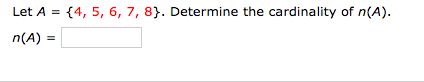# {4, 5, 6, 7, 8}. Determine the cardinality of n(A). n(A) = Let A Translate "s less 12.2 is 36.1" into an algebraic equation. Do not solve the equation.

Questionhelp_outlineImage Transcriptionclose{4, 5, 6, 7, 8}. Determine the cardinality of n(A). n(A) = Let A fullscreenhelp_outlineImage TranscriptioncloseTranslate "s less 12.2 is 36.1" into an algebraic equation. Do not solve the equation. fullscreen